##### Postgres Modulo Function With Examples

When we divide two numbers, sometimes the given numbers are fully divided, while sometimes the given numbers are not equally divided. In such a case, we get a remainder. In Postgres, the DIV() function performs the division on the input numbers and retrieves the quotient. What if the user wants the remainder instead of a quotient? How to deal with such cases? Well! Nothing to worry about! Because postgres facilitates us with a MOD() function that retrieves the remainder.

This post explains various use cases of the MOD() function via practical demonstration.

How Does the MOD() Function Work in Postgres?

PostgreSQL provides a built-in math function named MOD() that accepts numeric values as arguments, performs division, and retrieves the remainder after division. The return type of the MOD() relies on the data type of the input values.

Example 1: MOD() Function With Integer Values

We pass the integer values to the MOD() function in the following query:

`SELECT MOD(125, 12) As Remainder;`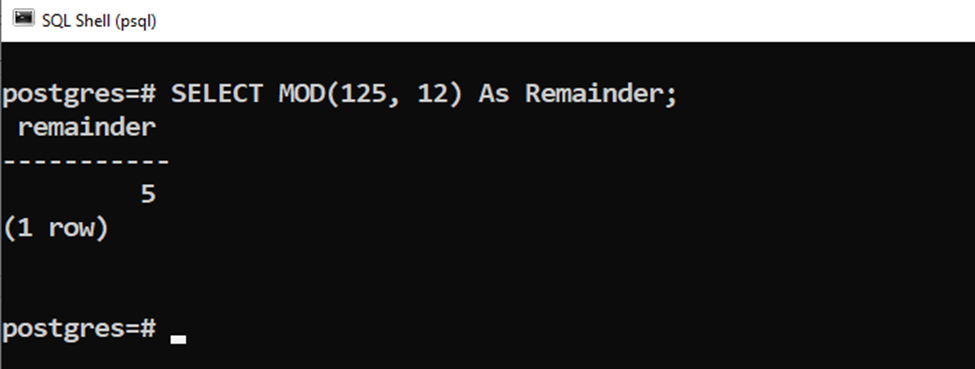The MOD() function retrieves an integer as a remainder.

Example 2: MOD() Function With Float Values

We pass the floating point values to the MOD() function in the below snippet:

`SELECT MOD(125.25, 12.70) As Remainder;`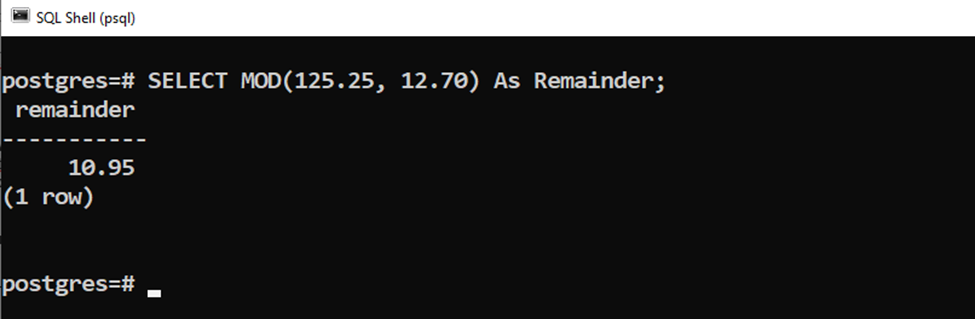The MOD() function retrieves a float value as a remainder.

Example 3: MOD() Function With Negative Numerator

We pass a negative numerator to the MOD() function in the below snippet:

`SELECT MOD(-125, 12) As Remainder;`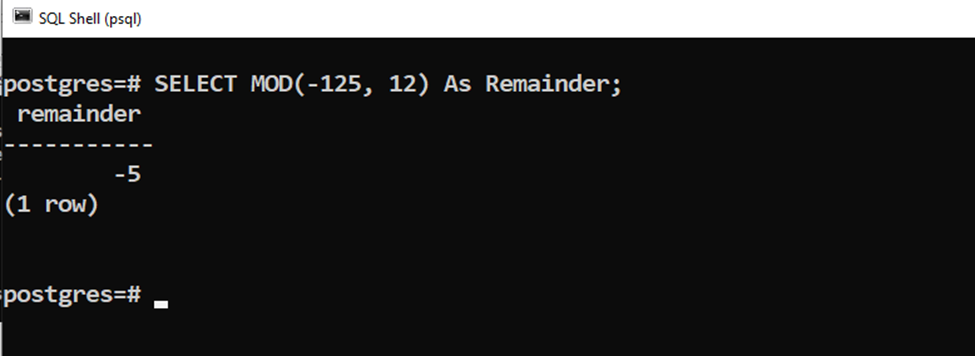The MOD() function retrieves a negative remainder when a negative numerator is passed to the MOD() function.

Example 4: MOD() Function With Negative Denominator

In the below example, we pass a negative denominator to the MOD() function:

`SELECT MOD(125, -12) As Remainder;`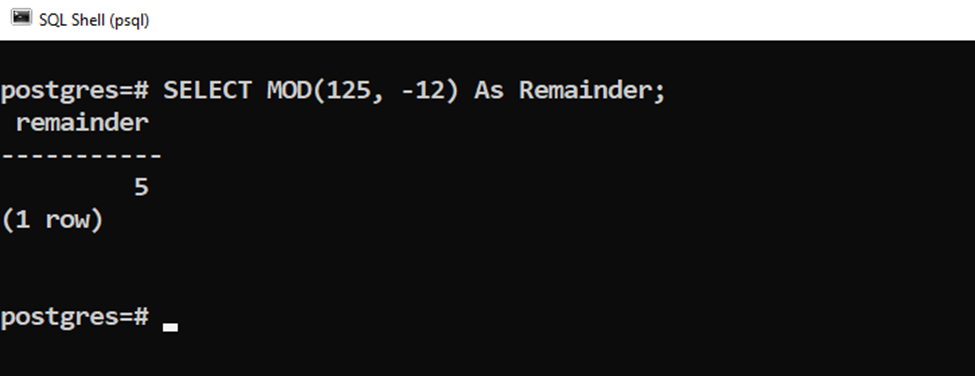The MOD() function retrieves a positive remainder when a negative denominator is passed to the MOD() function.

Example 5: MOD() Function With Both Negative Values

In the following code snippet, we pass a negative numerator and negative denominator to the MOD() function:

`SELECT MOD(-125, -12) As Remainder;`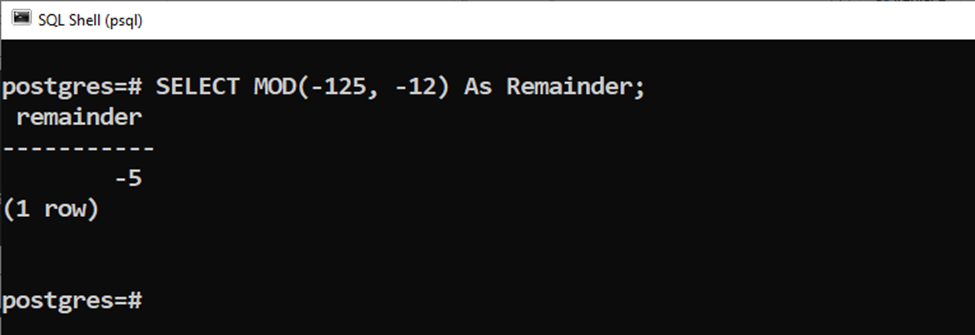The MOD() function retrieves a negative numerator.

Note: from the above examples, we can conclude that the remainder’s sign depends on the numerator. If the numerator is positive, we will get a positive remainder; if the numerator is negative, we will get a negative remainder.

Example 6: How to Use the MOD() Function With Postgres Tables?

We have a sample table named “example_data” that contains the following data:

`SELECT * FROM example_data;`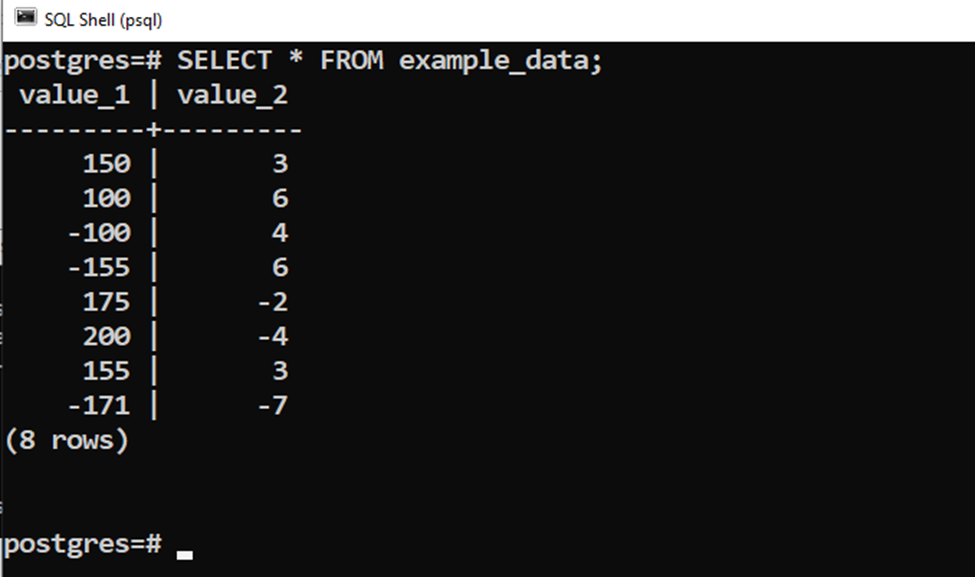The below snippet demonstrates the usage of the MOD() function on the table’s data:

```SELECT value_1, value_2, MOD(value_1, value_2)
FROM example_data;```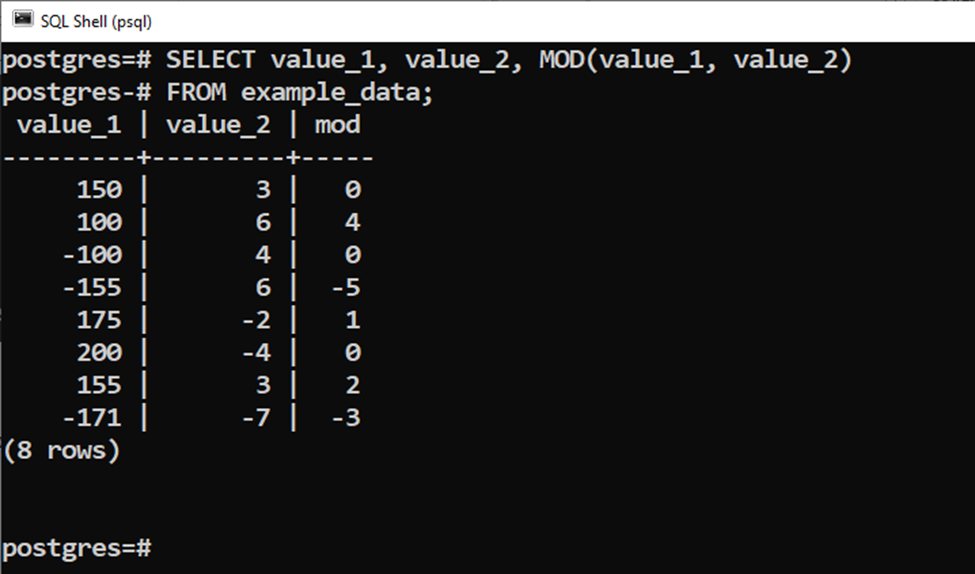This way, you can use the MOD() function to find the "remainder" of two values.

Conclusion

PostgreSQL provides a built-in math function named MOD() that accepts numeric values as arguments, performs division, and retrieves the remainder after division. The return type of the MOD() relies on the data type of the input values. The remainder’s sign depends on the numerator. If the numerator is positive, we will get a positive remainder; if the numerator is negative, we will get a negative remainder. This post used practical examples to explain a comprehensive guide on the MOD() function.